# Part Three: Mythic Theory of Everything

`Usually, there is a fine line between success and failure,But sometimes, there is no line at all.`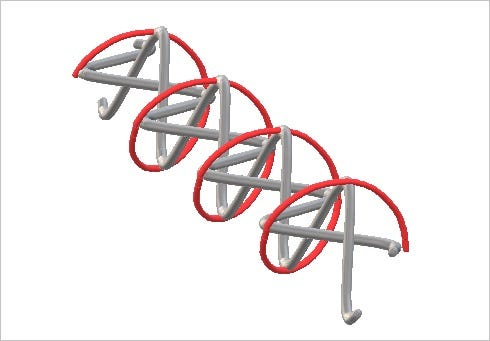Circular Wave with Horizontal and Vertical Components

## Foreword

The Mythic Theory of Everything (MToE) is a fabricated theory created as a parody of modern physics. For some it might be seen as pseudo-science, although at this time there is no advocate presenting it as actually true. I freely agree that it is nonsense and it should not be taken seriously. In that capacity, it can never be anything more than just junk science. Ironically, it demands explicit adherence to the rules that it is built on, so it makes a great thought experiment. This essay addresses how Mass Energy Equivalence looks when viewed with the MToE.

This article is part of the series called the Mythic Theory of Everything. If you view my About data, a list of all the other articles can be seen.

## Chapter 1 — Background

Do not under any circumstance study the MToE as actually theory. Keep in mind that the proper use of the MToE is to educate from a devil’s advocate position. For example, if I disagree with an aspect of modern physics, e.g. photons, rather than rant and rave about the subject and disavow the volumes of scientific literature, I simply ask the reader to explain why the MToE is wrong. Admittedly, the MToE concept is ridiculous, but it still demands thoughtful rebuttal to dismiss.

The first concept of the MToE states that giant filament loops fill up our universe. The filaments themselves are super strong, immensely long, very thin, and completely friction-less. These loops exist in a jumble that defines our universe. Other jumbles of filaments might well constitute other universes that we can not detect.

The second concept of the MToE states that all energy is transmitted though the filaments that make up the universe. As such, all treatment of electromagnetic phenomena must be viewed as actual waves and vibrations in these filaments.

The third concept states that mass is a stored form of energy when a loop is created in a one of these filaments. The loop can roll along the filament and represents an elemental particle. The loop also rotates around the filament to impart electromagnetic properties.

`Details of the original MToE can be found at:https://medium.com/@jbjo1956/f87b02ba8120?source=friends_link&sk=5ed0774234f81f32fd1fec47b5ee8c27An excellent article on the Mass–Energy Equivalence is found at:https://en.wikipedia.org/wiki/Mass–energy_equivalence 24 January 2022, at 22:21 (UTC)`

## Chapter 2 — Mass Energy Equivalence

If you involve yourself with modern physics (or have an associate or spouse who does) then you are probably familiar with Einstein’s famous equation:

E = mc²

In simplistic terms, the stuff in your cup that you are drinking right now has enough latent energy to level the building you are in and the surrounding neighborhood for many miles around. Fortunately for us, it is difficult to activate.

The MToE offers (in its nonsensical way) a visualization of physics. The concept of energy waves becomes actual waves in filaments. Graph the analogs and you can see the phenomena at work. For example, the diagram of the mass loop for an electron actually measures the diameter of an electron! From this I had a clever thought, measure the length of strands that make a mass loop and see if they are the same length as the strands that define an energy wave. One would expect them to be different and would finally let me demonstrate the false nature of the MToE.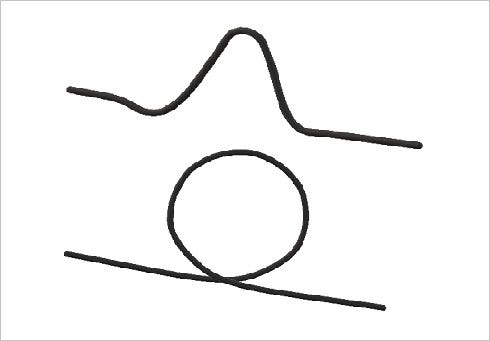MToE Representation of an Energy Wave and a Particle

We know the mass and energy equivalence of an electron.

`https://en.wikipedia.org/wiki/Electron_rest_mass 23 January 2022, at 16:33 (UTC)The electron rest mass... is the mass of a stationary electron, also known as the invariant mass of the electron. It is one of the fundamental constants of physics. It has a value of about 9.109×10−31 kilograms... equivalent to an energy of about 8.187×10−14 joules or about 0.5110 MeV.`

Sadly, we do not have a precise knowledge about the physical size of an electron, nor do we have any idea what the energy wave would look like. We can deduce the frequency and the corresponding wave length from the energy, but little more.

`https://en.wikipedia.org/wiki/Planck_constant 18 January 2022, at 15:04 (UTC)A photon’s energy is equal to its frequency multiplied by the Planck constant.`

If we are looking at waves in the ocean, the amplitude (height) determines the energy in the wave. This follows because a 20 foot high wave has the weight of 20 feet of water to push around. The problem with electromagnetic waves is that the amplitude part of the wave form does not store energy. Max Planck could probably tell you exactly why, but the fact that he used frequency and not amplitude in the formulation of his energy calculation tells us there is definitely a problem.

Another problem with amplitude may arise due to the polarization of the energy wave.

`https://en.wikipedia.org/wiki/Linear_polarization 26 December 2021, at 12:27 (UTC)https://en.wikipedia.org/wiki/Circular_polarization 11 January 2022, at 09:49 (UTC)`

In the MToE we can assume that the length of the curve between wavelengths is always the same for a given energy. This is, by the way, not a real world observation. Thus, in the MToE, as the polarization becomes less linear, the amplitude would be expected to shrink, while the wavelengths would stay the same.

By the way, if the MToE expects the filaments to be elastic, then changing the actual energy in the wave would definitely create different lengths. This is similar to looking at the length of a rubber band as it is stretched by attaching different weights.

With the MToE not having any decent mathematical solution, the idea of comparing lengths to invalidate the theory can be abandoned.

## Chapter 3 — Mass Conversion to Energy

If we exam the circular polarized wave profile, we discover another way to compare the MToE wave and particle configurations.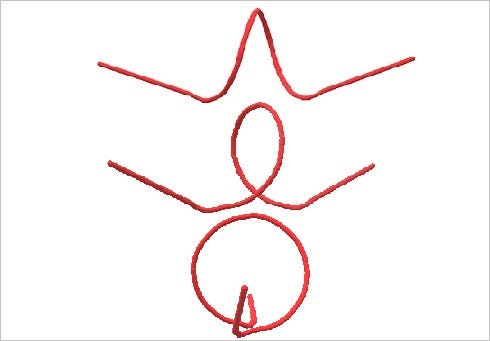Circular Wave Profile as it Rotates through 90 degrees

In the top level of the diagram, we see the side view of an energy wave pulse. It has the familiar sine wave we expect. From the side, we can not determine the polarity, because the linear and circular polarities look the same. In the middle level, we see the wave pulse rotated about 45 degrees. It is now clearly has circular polarity. In the bottom level, we see the pulse as a circle.

The outline of the mass loop is clearly defined at the center of the circularized wave profile. Are they actually the same size? You be the judge.

If we accept it as true, then the MToE predicts that a particle converts to a circular polarized energy wave. So then all we need to do is measure the polarity of a gamma ray to support or disprove the MToE? Imagine firing a gamma ray (or a burst of gamma rays) into a conventional mono-filament polarity filter. Or not… Actually, there are detectors that will do this, but they are complicated animals.

`If you want a career in gamma ray physics, you might consider this:https://arxiv.org/pdf/2109.03286.pdf GRB Polarization: A Unique Probe of GRB PhysicsRamandeep Gill, Merlin Kole, and Jonathan Granot, © 2019`

Regardless, the MToE shows that a gamma ray converts to a particle by being flattened and a particle converts to a gamma ray by popping out of shape. It is an intriguing transformation and it is remarkably simple as these things go. (If only it were true in the real world…)

By the way, gamma rays do actually produce particles in a real process call “Pair Production.” The MToE process is fictional.

`https://en.wikipedia.org/wiki/Gamma_ray 6 February 2022, at 18:47 (UTC)Pair production: This becomes possible with gamma energies exceeding 1.02 MeV … By interaction with the electric field of a nucleus, the energy of the incident photon is converted into the mass of an electron-positron pair.`

In a related note, the MToE does make an interesting prediction. If the gamma ray core can not collapse into a circle, but collapses to a wide or tall ellipse, it is unlikely a particle will form. If this were actually true, it might explain quantum properties of particles in the sense that particles do not come in a continuous series, but tend to be quite unique animals.

## Chapter 4 — Just for Fun

We are not doing anything important here, so lets have a little fun.

`Frequency – Wavelength – Energy Converterhttps://www2.chemistry.msu.edu/faculty/reusch/virttxtjml/cnvcalc.htm`

If an electron has .5110 MeV mass then we should be able to say the energy wave also has .5110 MeV or 511000 eV. Using the converter, (so I do not have to look stupid if I flub the math), we get a wavelength of 2.4263e-12 m.

Actually, this is the Compton wavelength. I did not really need to calculate it!

`https://en.wikipedia.org/wiki/Compton_wavelength The Compton wavelength of a particle is equal to the wavelength of a photon whose energy is the same as the mass of that particle.… the Compton wavelength of the electron is 2.42631023867(73)×10^-12 m. Other particles have different Compton wavelengths.`

There is a rule of thumb that the aperture that a wave can pass through is between 50% and 100% of the wavelength. (Rule of thumb… we expect people to have thumbs, but they are still people even if they do not.)

`Sorry, this rule is inferred from the wavelength size and the resolution of the photo-lithography.  No one actual said it, but here is the data.https://www.anandtech.com/show/8223/an-introduction-to-semiconductor-physics-technology-and-industry/4An Introduction to Semiconductor Physics, Technology, and Industryby Joshua Ho on October 9, 2014 3:00 PM EST`

So we deduce that an electron is between 1.2e-12 m and 2.4e-12 m in diameter.

`In actuality, according to this reference:https://journals.aps.org/pr/abstract/10.1103/PhysRev.14.20The Size and Shape of the ElectronArthur H. ComptonPhys. Rev. 14, 20 – Published 1 July 1919It is concluded that the diameter of the electron is comparable in magnitude with the wave-length of the shortest γ-rays. Using the best available values for the wave-length and the scattering by matter of hard X-rays and γ-rays, the radius of the electron is estimated as about 2 × 10^−10 cm.  [i.e. 2e-10 cm]`

Converting cm to m gives 2e-12 m and doubling to get the diameter gives 4e-12 m. Thus, our value is 1.6e-12 m away from this reference value.

`Of course, there is the official reference:https://en.wikipedia.org/wiki/Electron 22 January 2022, at 14:02 (UTC)The issue of the radius of the electron is a challenging problem of modern theoretical physics. The admission of the hypothesis of a finite radius of the electron is incompatible to the premises of the theory of relativity. On the other hand, a point-like electron (zero radius) generates serious mathematical difficulties due to the self-energy of the electron tending to infinity. Observation of a single electron in a Penning trap suggests the upper limit of the particle's radius to be 10^−22 meters. The upper bound of the electron radius of 10^−18 meters can be derived using the uncertainty relation in energy. There is also a physical constant called the "classical electron radius", with the much larger value of 2.8179×10^−15 m, greater than the radius of the proton. However, the terminology comes from a simplistic calculation that ignores the effects of quantum mechanics; in reality, the so-called classical electron radius has little to do with the true fundamental structure of the electron.`

In this case our calculation is at least a thousand times larger than any recognized values. So who is to be believed?

There may well be some actual physics involved. Is it possible that energy can only be constrained in a minimum limited space? The MToE works under the principle of absolute constraint and modern physics uses the hands off energy density principle, i.e. points can hold unlimited energy or it can be spread over broad areas. Maybe the truth is somewhere in between.

## Chapter 5 — Multi-Loop Particles

Some of you may see the obvious problem and think “Let’s take the MToE off the table. Protons have about two-thousand times more mass than electrons, so by the MToE they should be considerably bigger. They are in fact smaller.”

Perhaps being composed of three particles and numerous gluons, they have learned to live in smaller and more crowded houses. Or… there may be a more logical answer: Multi-Loop Particles.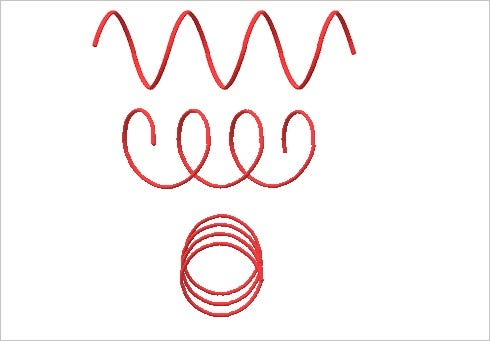Circular Wave Profile of a Full Wave as it Rotates through 90 degrees

The MToE has used wave pulses up to this point, but there is no reason we could not use a full wave. In the top level of the diagram, we see the side view of a full energy wave. It has the familiar sine wave we expect. From the side, we can not determine the polarity, because the linear and circular polarities look the same. In the middle level, we see the full wave rotated about 45 degrees. It is now clearly has circular polarity. In the bottom level, we see the wave as a series of circles.Multi-Loop Particle from Collapsed Circular Wave

Again, the MToE shows that a full gamma ray converts to a string of particles when flattened and a particle fountain of gamma rays when popped out of shape. It is again an intriguing transformation.

`https://en.wikipedia.org/wiki/Jet_(particle_physics) 8 October 2021, at 13:18 (UTC)Nice Illustration:https://profmattstrassler.files.wordpress.com/2012/03/bjet_vs_ujet.png?w=770Illustration from:https://profmattstrassler.com/articles-and-posts/particle-physics-basics/the-known-apparently-elementary-particles/jets-the-manifestation-of-quarks-and-gluons/b-tagging-identifying-jets-from-bottom-quarks/B-tagging: Identifying Jets from Bottom QuarksMatt Strassler [March 7th, 2012]`

This is an actual phenomena, but it does not mean the MToE is correct. It is just a coincidence.

In any case, the multi-loop particle does not have to be as wide as the single pulse loop of the electron to have more mass and consequently carry more energy. Thus the quarks in the proton could actually fit in a smaller house.

## Chapter 6 — The Paradox

The paradox of energy waves and the aperture size that they manifest is that as the energy goes up the wave-length decreases. This creates a paradox for the MToE when the energy wave is converted to a particle, because the MToE predicts that the particle must be larger as the energy increases! Do not worry about the health of the MToE though. If we use the De Broglie Wavelength for an electron we are back in the vicinity of our original prediction for the size of an electron, 2.4e-12 m.

`https://www.thoughtco.com/de-broglie-wavelength-example-problem-609472By Todd Helmenstine, Updated on June 05, 2019Answer:  The wavelength of an electron moving 5.31 x 10^6 m/sec is 1.37 x 10^-10 m or 1.37 Å.`

Although one’s first thought might be, “Well it just another wave-length calculation. It should stay close to the known energy of an electron in any case,” that is not necessarily the way the MToE works.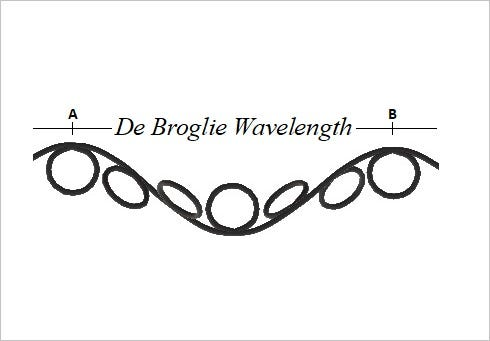De Broglie Wavelength of a Particle Wave

The MToE representation of a particle wave is a large rotating sine wave with a small counter spinning inner loop.

My next segment on Wave Particle Duality explores this concept further. —— Junk Science Version of Wave Particle Duality

## Chapter 7— Conclusions

The beauty of working with junk science like the MToE is that I do not have to spend time defending the propositions that it creates. If it is proven wrong, I shrug my shoulders and say “Ok.” If it should be proven correct, I shrug my shoulders and say “It was a lucky guess.” To be frank though, I would be lying if I did not acknowledge that I do find some aspects of this essay disturbing.

--

--